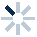# 3.7.1 必修1 项目七 设计算法实现用数学公式计算 学案11. 1、数据数值分类：整型（int）和浮点型（float）,整型int包括{..-3,-2,-1,0,1,2,3...},浮点数是带小数的数，如23.56
2、认识python各类运算符：A. 18//4=4
B. －18//4=-5
C. 2**4=16
D. 10%2=5
2.Python语言中通过for语句来实现确定次数循环。

for <循环控制变量> in <遍历结构>:

<语句块>

1、用for语句实现求50!的程序:2、Python 编程中 while 语句用于实现不确定次数的循环，在某条件下，循环执行某段程序，以处理需要重复处理的相同任务。

while 判断条件 ：
执行语句……

2、用while语句来实现求50!的程序：1、分析问题

2、设计算法：3、设计程序：*
3. 课外拓广：math中的常用函数A.math.sqrt( 16 )=8
B.math.pow( 2,10)=1024
C.math.fmod(16,6)=2.0
D.math.factorial(4)=20
4. 活动7.1 使用python实现沃利斯公式和梅钦公式计算圆周率：*